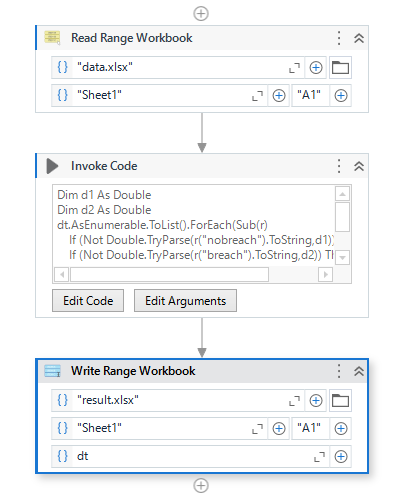# Add two column value without loop

Can anyone idea about LINQ how we add Column (Nobreach), column (Breach) and save in data will Grand Total column.

have you tried with Write cell activity?

Regards
Gokul

Try this:

``````dataTable = (From row In dataTable.AsEnumerable()
Let firstValue = row.Field(Of Integer)("Nobreach")
Let secondValue = row.Field(Of Integer)("Breached")
Select row.Field(Of Integer)("Grand Total") = firstValue + secondValue).CopyToDataTable()
``````

Hope it helps.

I have 15k records so its take time while using write cell activity within the loops.

Can you try with this LINQ Expression?

``````dtWithGrandTotal.AsEnumerable().ToList().ForEach(
Sub(row)
Dim noBreachValue As Double = If(Double.TryParse(row.Field(Of String)("NoBreach"), 0.0), Double.Parse(row.Field(Of String)("NoBreach")), 0.0)
Dim breachValue As Double = If(Double.TryParse(row.Field(Of String)("Breach"), 0.0), Double.Parse(row.Field(Of String)("Breach")), 0.0)

' Calculate the Grand Total and set it in the new column
row.SetField("Grand Total", noBreachValue + breachValue)
End Sub
)
``````

Regards
Gokul

It will throw this type error which I mention below.

Hi,

How about using AutoFill as the following sample?

Sample
Sample20231004-6L.zip (8.5 KB)

Regards,

Actually, challenge is I don’t have excel in that machine.

@Anish_Kumar2

Clone the original DataTable structure first
Assign: `updatedDataTable = dataTable.Clone()`

Assign:

``````updatedDataTable = (From row In dataTable.AsEnumerable()
Let firstValue = row.Field(Of Integer)("Nobreach")
Let secondValue = row.Field(Of Integer)("Breached")
Let thirdValue = firstValue + secondValue
``````

@Anish_Kumar2 Try this in invoke code with your datatable as IO argument.

`io_DataTable.asenumerable.tolist.foreach(Sub(row) row(3)= (Cdbl(row(1))+Cdbl(row(2))).ToString)`

1 Like

Can you try the following sample?``````Dim d1 As Double
Dim d2 As Double
dt.AsEnumerable.ToList().ForEach(Sub(r)
If (Not Double.TryParse(r("nobreach").ToString,d1)) Then d1 =0
If (Not Double.TryParse(r("breach").ToString,d2)) Then d2 =0
r("grand total")=d1+d2
End Sub
)
``````

Sample20231004-6L (2).zip (8.4 KB)

Regards,

Show this ErrorAssign: Unable to cast object of type ‘System.String’ to type ‘System.Int32’.

I think the column has string values

``````updatedDataTable = (From row In dataTable.AsEnumerable()
Let firstValue = Integer.Parse(row.Field(Of String)("Nobreach"))
Let secondValue = Integer.Parse(row.Field(Of String)("Breached"))
Let thirdValue = firstValue + secondValue